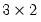Command Reference : Matrix Language Reference

 @filledmatrix
Create and fill matrix with scalar value.
Syntax: @filledmatrix(n1, n2, n3)
n1: integer
n2: integer
n3: number
Return: matrix
Returns a matrix with n1 rows and n2 columns, where each element contains the value n3.
Examples
matrix(3, 2) m1
m1 = pi
matrix m2 = @filledmatrix(3, 2, @pi)
creatematrices M1 and M2 where each element is set to Pi. The function allows for the creation and initialization of the matrix using a single command.
Cross-references
See @fill for creating a vector using multiple values and @unvec for creating a sym from a vector.